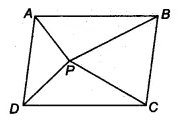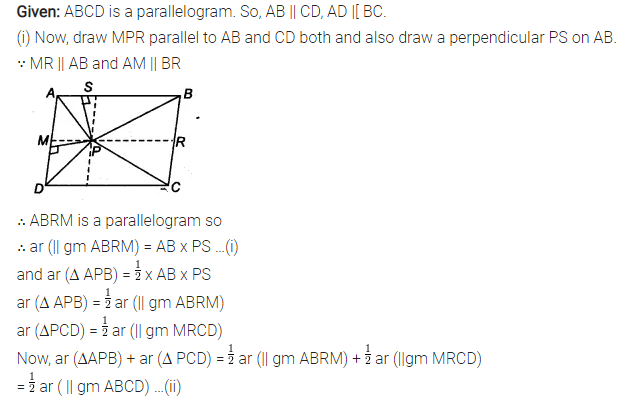# In figure, P is a point in the interior of a parallelogram ABCD

In figure, P is a point in the interior of a parallelogram ABCD. Show that
(i) ar (APB) + ar (PCD) = \frac { 1 }{ 2 } ar (ABCD)
(ii) ar (APD) + ar(PBC) = ar (APB) + ar (PCD)